# Tag Archives: Renglish

There are now more than 10,000 R packages available from CRAN, much more if you include those available only on github. So, to be honest, it become difficult to know all of them. But sometimes, you discover a nice function in one of them, and that is really awesome. Consider for instance some (standard) censored lifetime data,

```n=10000 idx=sample(1:4,size=n,replace=TRUE) pd=LETTERS[idx] lambda=1+(idx-1)/3 t=rexp(n,lambda) x=rexp(n) c=t>x y=pmin(t,x) df=data.frame(time=y,status=c,product=pd)```

(yes, I will generate them here). Consider Kaplan-Meier estimator of the survival function,

```library(survival) km.base = survfit( Surv(time,status) ~ 1  , data = df ) plot(km.base)```This week end, Anat (currently finishing the Data Science for Actuaries program) made me discover a nice R function, to add information to that graph (well, not that graph, since it will be a ggplot version, but the same survival distribution plot)

```library(ggplot2) library(survminer) ggsurvplot(km.base, main = "", color = "blue" , censor = FALSE, xlim = c(0,3), risk.table = TRUE , risk.table.col = "blue" , risk.table.height = 0.2, risk.table.title = "" , legend.labs = "All" , legend.title = "" , break.time.by = 1, xlab = "" , ylab = "")```This is more interesting when we have different lifetimes
``` km.prod = survfit( Surv(time,status) ~ product  , data = df ) ggsurvplot(km.prod, main = "", censor = FALSE, xlim = c(0,3), risk.table = TRUE , risk.table.col = "strata" , risk.table.height = 0.3, risk.table.title = "" , legend.labs = LETTERS[1:4] , legend.title = "" , break.time.by = 1, xlab = "" , ylab = "")```or, with a different time granularity

`ggsurvplot(km.prod, main = "", censor = FALSE, xlim = c(0,3), risk.table = TRUE , risk.table.col = "strata" , risk.table.height = 0.3, risk.table.title = "" , legend.labs = LETTERS[1:4] , legend.title = "" , break.time.by = .5, xlab = "" , ylab = "")`Nice, isn’t it?

# Advanced Econometrics: Quantiles and Expectiles

Next Thursday, I will give the fourth lecture of the PhD course on advanced tools for econometrics, on quantile and expectile regressions. Slides are available online.

# The U.S. Has Been At War 222 Out of 239 Years

This morning, I discovered an interesting statistic, “America Has Been At War 93% of the Time – 222 Out of 239 Years – Since 1776“,  i.e. the U.S. has only been at peace for less than 20 years total since its birth. I wanted to check, get a better understanding and look at other countries in the world.

As always, we can try to extract information from wikipedia, since there are pages dedicated to that information

```url="https://en.wikipedia.org/wiki/List_of_wars_involving_the_United_States" download.file(url,destfile = "warUS.html") url="https://en.wikipedia.org/wiki/List_of_wars_involving_France" download.file(url,destfile = "warFR.html") url="https://fr.wikipedia.org/wiki/Liste_des_guerres_de_la_France#Premi.C3.A8re_R.C3.A9publique" download.file(url,destfile = "guerre.html") url="https://en.wikipedia.org/wiki/List_of_wars_involving_Canada" download.file(url,destfile = "warCAN.html")```

If we look at the US page, there are tables, so it should be easy to extract it. For instance,Even if the war did last 1 day, we will say that the US were at war in 1811. The information we want to confirm can be “there were 21 full years – from Jan 1st till Dec 31st – where the US were not at war, once, during those years“. From the row above, we can claim that the US were at war in 1811. Most of the time, we haveI.e. there is a beginning (here 1775) and an end (1783). So here, the US are said to be at war in 1775, 1776, 1777, 1778, 1779, 1780, 1781, 1782, 1783. To extract the information, we look for regular expressions in the first column, with number, on 4 digits.Well, sometimes it can be a bit tricky, since we have 3 dates, 1941, 1945 and (in the legend) 1944. But if we consider the minimal and the maximal dates, we have our range of dates.

Now that we we how to extract information, let’s do it. The code will be
``` library(stringr) ext_date=function(x){ dates12="[0-9]{4}" #grep(pattern = dates2, x = col1) L=str_extract_all(as.character(x),dates12) return_L=list() if(length(L)>0){ for(j in 1:length(L)) if(length(L[[j]])==1) return_L[[j]]=as.numeric(L[[j]]) if(length(L[[j]])>=2) return_L[[j]]=seq(min(as.numeric(L[[j]])),max((as.numeric(L[[j]])))) } return(return_L)}```

For the US, we get the following years

```library(XML) tables=readHTMLTable("warUS.html") list_dates=list() for(i in 1:length(tables)){ if(!is.null(dim(tables[[i]]))){ if(ncol(tables[[i]])>1){ col1=tables[[i]][,1] list_dates[[i]]=lapply(col1,ext_date) } }} d=unique(unlist(list_dates))```(red means at war, while green means no-war) and indeed,

```> length(d)  222```

there were 222 years with war.  Now, what about another country. Like France. Here I use the French wiki page, since information is not in tables in the English one.

```tables=readHTMLTable("guerre.html") list_dates=list() for(i in 1:length(tables)){ if(!is.null(dim(tables[[i]]))){ if(ncol(tables[[i]])>1){ col1=tables[[i]][,1] col2=tables[[i]][,2] col12=paste(col1,col2) list_dates[[i]]=lapply(col12,ext_date) } }} d=unique(unlist(list_dates))```

On the same period of time (starting in 1775), France was also on war most of the time.Less than the US, but still: 185 years with war,

```> length(d[d>=1775])  185```

And on a longer period of time? Why not start, say, around the Hundred Years’s War,meaning that since 1337, there were (only) 174 years without a single war where France was involved.

Let’s try another one. Like Canada,

```tables=readHTMLTable("warCAN.html") list_dates=list() for(i in 1:length(tables)){ if(!is.null(dim(tables[[i]]))){ if(ncol(tables[[i]])>1){ col1=tables[[i]][,1] list_dates[[i]]=lapply(col1,ext_date) } }} d=unique(unlist(list_dates))```Guess what… there’s a lot of green on that graph. Surprised?

It is now very easy to read (automatically) some text that can be found in a pdf file. For instance, consider the program of the conference we had yesterday – and today – in Rennes```> library(pdftools) > scan_pdf <- pdf_text("http://crem.univ-rennes1.fr/Documents/Docs_sem_divers/2017_03_10-11_JJD/JDD_prog.pdf") > cat(scan_pdf) Journées Jeunes Docteurs Programme du jeudi 9 mars 2017 Faculty of Economics - Rennes - Amphi Henri Krier 9h- 9h30 - Accueil 9h30-10h15 :      Présentation du CREM, de la faculté et des activités de recherche liées du ou laboratoire 10h15-10h50 :     Emmanuel LORENZON (Université de Bordeaux, GREThA) Collusion with a rent seeking agency in sponsored search auctions 10h50-11h25 :     Julien BERTHOUMIEU (Université de Bordeaux, GREThA) The Impact of “At-the-Border” and “Behind-the-Border” Policies on Cost-Reducing Research and Development Co-écrit avec Antoine Bouët```

(etc). As you can see, it is working well, even in French, where we have those weird letters (with accents). Here, it is working well because the pdf is vectorized, i.e. it was generated properly, by open office.

But sometimes, we can have only a scanned version of a letteror just a picture with some typed text. I will not mention hand-writing because it is much more complex.

The other day, my friend Fleur did show me a picture, and some very simple lines of code,```> library('tesseract') > pic1="http://freakonometrics.hypotheses.org/files/2017/03/pic1.png" > text_fr <- ocr(pic1, engine = tesseract("fra")) > cat(text_fr) Près de 14.400 décès```

```Si [épidémie de grippe est un phénomène récurrent. celle de 2016—2017 présente plusieurs spéciﬁcités. outre sa virulence : une survenue plus précoce que d‘habitude. une activité modérée en médecine ambulatoire. mais un impact fort en milieu hospitalier.```

It looks like we’ve be able to extract typed text from a picture ! I want to check. I have to admit, first of all that installation on a linux machine is tricky: one has to install first leptonica, and then follow some guidelines to install tesseract (see also Artem‘s advices). It took me some time, but I’ve been able to install the package.

The first important step, it to train the algorithm with some texts in French (because it is in French in my picture)

```> library('tesseract') > tesseract_download("fra")```

Then, I did try with the picture that Fleur did send me (the picture was inserted in the core of the message)```> pic2="http://freakonometrics.hypotheses.org/files/2017/03/pic2.png" > text_fr <- ocr(pic2, engine = tesseract("fra")) > cat(text_fr) Près de 14400 decès```

```s. mm….agw«… ………«…m …… a……u…u Dhs—ur; ;pmum…. ;: u…… … W»: »… w…q…na… … …… …… ………u…_ …… mm…… …… nwm/u```

```… … mm…—mg…»— sa…… su a.…….… : :mmræwesdæ ; ; m…decnﬂwtülﬂws WWW… un»… M on m…… . … … .. m...… wma: .,… … V, …… … …;………yg…gn… …… pe- le…samemeuuœwpwv m… mum```

Clearly, something went wrong here. When I got that output, I thought that I did not train properly the function. But it was not the answer. As described in that post (in French) it is necessary to have a clean picture, to read it properlyAnd actually, if we zoom in our picture – the first one, used by Fleur, to show me that package – we havewhile for the second one – with a lower resolution – we haveIt is necessary to have a scan of a typed text with high resolution… And you have to admit that it is awesome….

The good thing is that I have to work with a judge, in France, to assess quality of experts. And since most of the reports are typed, and then scanned, I am glad to have such a function. I just have to make sure that the resolution is high enough…

On Thursday, March 23rd, I will give the third lecture of the PhD course on advanced tools for econometrics, on model selection and variable selection, where we will focus on ridge and lasso regressions . Slides are available online.

The first part was on on Nonlinearities in Econometric models, and the second one on Simulations.

# Ruin probability and infinite time

A couple of weeks ago, I had a discussion with a practitioner, working in some financial company, about ruin, and infinite time. And it reminded me a weird result. Well, not a weird result, but a result I found disturbing, at first, when I was a student (that I rediscovered with the eyes of someone dealing with computational issues, seeing here a difficult theoretical question). Consider a simple ruin problem. A player has wealth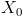. Then he flips a coin: tails he has a gain of 1, heads he experiences a loss of 1. At time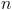, his wealth is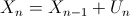where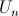is associated to theth coin:is equal to 1 with probability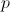(tails), and -1 with probability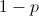(heads). It is also possible to write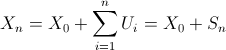where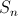can be interpreted as the net gain of the player. In order to get a good understanding of results that can be obtained. Assumeto be given. Let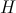denote the number of heads and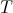the number of tails. Then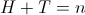, while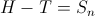. Let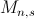denote the number of paths to go from point A (wealth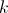at time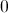) to point B (wealth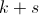at time). Note that this is a Markovian problem, that can be modeled using Markov chainsBut here, we will focus on combinatorial results. Hence,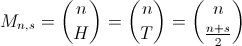In order to derive probabilities to reach, let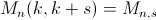denote the number of paths going from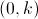to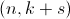. And let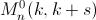denote the number of paths going fromtothat do reachat some point betweenand. Using a simple reflexion property, then ifandare positive,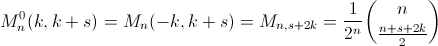Based on those reflexions, two results can be derived (focusing on probability, instead of counting paths). First, we can obtain that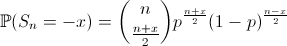(given that n and x have the same parity). The second result we can obtain is that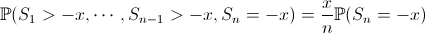Based on those two expressions, if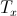denotes the first time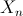become null, given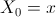,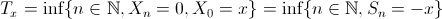then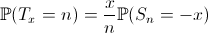This can be computed easily,

```> x=10
> p=.55
> ProbN=function(n){
+ pb=0
+ if(abs(n-x) %% 2 == 0)
+ pb=x/n*choose(n,(n+x)/2)*(1-p)^((n+x)/2)*(p)^((n-x)/2)
+ return(pb)}
> plot(Vectorize(ProbN)(1:1000),type="s")```That looks nice… But if we look closer, we can wonder what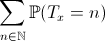would be ? Since we have the distribution of a probabilty measure, we might expect one. But here

```> sum(Vectorize(ProbN)(1:1000))
 0.134385```And this is not due to calculation mistakes that we do not get 1 here. Actually, we should write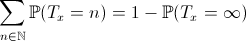which might be interpreted as the probability of ruin, starting from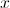, that we denote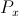from now on. The term on the left can be approximated using monte-carlo simulations

```> p=.55
> x=10
> m=1000
> simul=10000
> S=sample(c(-1,1),size=m*simul,replace=TRUE,prob=c(1-p,p))
> MS=matrix(S,simul,m)
> for(k in 2:m) MS[,k]=MS[,k]+MS[,k-1]
> T0=function(vm) which(vm<=(-x))
> MTmin=apply(MS,1,T0)
> mean(is.na(MTmin)==FALSE)
 0.1328```

To check the validity of the relationship above, a simple (theoretical) recursive formula can be derived for the term on the right (ruin probability), namely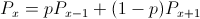with a boundary conditions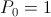, and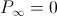. Then is comes that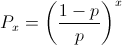Note that it might be tricky to check using monte carlo simulation… since we cannot have an infinite number of runs. And we’re dealing precisely with things that do occur when time is infinite. Actually, we can still check convergence, considering an upper limit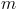for the number of runs, and then lettinggo to infinity. Note that an explicit formula can then be derived (using additional border condition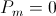)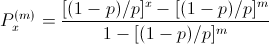Using the following code, it is possible to calculate ruin probability, in order to estimate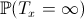.

```> MSmin=apply(MS,1,min)
> mean(MSmin<=(-x))
 0.1328
> (((1-p)/p)^x-((1-p)/p)^m)/(1-((1-p)/p)^m)
 0.1344306```

The following graph shows the evolution of ruin probability as a function of initial wealth (with monte carlo simulation, with a fixed horizon – including a confidence interval – versus the analytical expression)Hence, with stopping times, one should remember that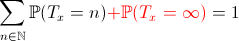and that those two terms can be approximated simply using simulations or standard approximations.

# Basics on Markov Chain (for parents)

Markov chains is a very interesting and powerful tool. Especially for parents. Because if you think about it quickly, most of the games our kids are playing at are Markovian. For instance, snakes and ladders…It is extremely easy to write down the transition matrix, one just need to define all snakes and ladders. For the one above, we have,

```n=100
M=matrix(0,n+1,n+1+6)
rownames(M)=0:n
colnames(M)=0:(n+6)
for(i in 1:6){diag(M[,(i+1):(i+1+n)])=1/6}
M[,n+1]=apply(M[,(n+1):(n+1+6)],1,sum)
M=M[,1:(n+1)]
starting=c(4,9,17,20,28,40,51,54,62,
64,63,71,93,95,92)
ending  =c(14,31,7,38,84,59,67,34,19,
60,81,91,73,75,78)
for(i in 1:length(starting)){
v=M[,starting[i]+1]
ind=which(v>0)
M[ind,starting[i]+1]=0
M[ind,ending[i]+1]=M[ind,ending[i]+1]+v[ind]}```

So, why is it important to have a Markov Chain ? Because, once you’ve noticed that you had a Markov Chain game, you can derive anything you want. For instance, you can get the distribution after some turns,

```powermat=function(P,h){
Ph=P
if(h>1){
for(k in 2:h){
Ph=Ph%*%P}}
return(Ph)}
initial=c(1,rep(0,n))
COLOR=rev(heat.colors(101))
u=1:sqrt(n)
boxes=data.frame(
index=1:n,
ord=rep(u,each=sqrt(n)),
abs=rep(c(u,rev(u)),sqrt(n)/2))
position=function(h=1){
D=initial%*%powermat(M,h)
plot(0:10,0:10,col="white",axes=FALSE,
xlab="",ylab="",main=paste("Position after",h,"turns"))
segments(0:10,rep(0,11),0:10,rep(10,11))
segments(rep(0,11),0:10,rep(10,11),0:10)
for(i in 1:n){
polygon(boxes\$abs[i]-c(0,0,1,1),
boxes\$ord[i]-c(0,1,1,0),
col=COLOR[min(1+trunc(500*D[i+1]),101)],
border=NA)}
text(boxes\$abs-.5,boxes\$ord-.5,
boxes\$index,cex=.7)
segments(c(0,10),rep(0,2),c(0,10),rep(10,2))
segments(rep(0,2),c(0,10),rep(10,2),c(0,10))}```

Here, we have the following (note that I assume that once 100 is reached, the game is over)Assume for instance, that after 10 turns, your daughter accidentally drops her pawn out of the game. Here is the theoretical (unconditional) position of her pawn after 10 turns,so, if she claims she was either on 58, 59 or 60, here are the theoretical probabilities to be in each cell after 10 turns,

```> h=10
> (initial%*%powermat(M,h))[59:61]/
+ sum((initial%*%powermat(M,h))[59:61])
 0.1597003 0.5168209 0.3234788```

i.e. it is more likely she was on 59 (60th cell of the vector since we start in 0). You can also look at the distribution of the number of turns (at first with only one player).

```distrib=initial%*%M
game=rep(NA,1000)
for(h in 1:length(game)){
game[h]=distrib[n+1]
distrib=distrib%*%M}
plot(1-game[1:200],type="l",lwd=2,col="red",
ylab="Probability to be still playing")```Once you have that survival distribution, you have the expected number of turns to finish the game,

```> sum(1-game)
 32.16499```

i.e. in 33 turns, on average, your daughter reaches the 100 cell. But in 50% of the games, it takes less than 29,

```> max(which(1-game>.5))
 29```

But assuming that you are playing with your daughter, and that the game is over once one player reaches the 100 cell, it is possible to get the survival distribution of the first time one of us reaches the 100 cell,

```plot((1-game[1:200])^2,type="l",lwd=2,col="blue",
ylab="Probability to be still playing (2 players)")```Here, the expected number of turns before ending the game is

```> sum((1-game)^2)
 23.40439```

And if you ask your son to join the game, the survival distribution function is

```plot((1-game[1:200])^3,type="l",lwd=2,col="purple",
ylab="Probability to be still playing (3 players)")```i.e. the expected number of turns before the end is now

```> sum((1-game)^3)
 20.02098```

# Short selling, volatility and bubblesYesterday, I wrote a post (in French) about short-selling in financial market since some journalists claimed that it was well-known that short -selling does increase volatility on financial market. Not only in French speaking journals actually, since we can read on http://www.forbes.com that  « in a market with restrictions on short-selling, volatility is reduced ». But things are not that simple. For instancehttp://www.optionsatoz.com/ explains it from a theoretical point of view. But we can also look at the data. For instance, we can compare the stock price of Air China, exchanged in Shanghai in blue (where short-selling is forbidden) and in Hong Kong in rouge (where short-selling is allowed), since @Igor gave me the tickers of those stocks

```library(tseries)
X<-get.hist.quote("0753.HK")
Y<-get.hist.quote("601111.SS")
plot(Y[,4],col="blue",ylim=c(0,30))
lines(X[,4],col="red")```But as @alea_ pointed out, one asset is expressed here in Yuans renminbi, and the other one in HK dollars. So I downloaded the exchange rate fromhttp://www.oanda.com/```Z=read.table("http://freakonometrics.blog.free.fr/public/
D=as.Date(as.character(Z\$date),"%d/%m/%y")
z=as.numeric(Z\$CNY.HKD)
plot(D,z,type="l")
X2=X[,4]
for(t in 1:length(X2)){ X2[t]=X2[t]*z[D==time(X2[t])]}
X2=X[,4]
plot(Y[,4],col="blue",ylim=c(0,30))
lines(X2,col="red")```Now both stocks are expressed in the same currency. To compare returns volatility, a first idea can be to use GARCH models,

```RX=diff(log(X2))
RY=diff(log(Y[,4]))
Xgarch = garch(as.numeric(RX))
SIGMAX=predict(Xgarch)
Ygarch = garch(as.numeric(RY))
SIGMAY=predict(Ygarch)
plot(time(Y)[-1],SIGMAY[,1],col="blue",type="l")
lines(time(X2)[-1],SIGMAX[,1],col="red")```But volatility is here too eratic. So an alternative can be to use exponentially-weighted moving averages, where simple recursive relationships are consideredor equivalentlyThe code is not great, but it is easy to understand,

```moy.ew=function(x,r){
m=rep(NA,length(x))
for(i in 1:length(x)){
m[i]=weighted.mean(x[1:i],
rev(r^(0:(i-1))))}
return(m)}
sd.ew=function(x,r,m){
sd=rep(NA,length(x))
for(i in 1:length(x)){
sd[i]=weighted.mean((x[1:i]-m[i])^2,
rev(r^(0:(i-1))))}
return(sd)}
q=.97
MX=moy.ew(RX,q)
SX=sd.ew(RX,q,MX)
MY=moy.ew(RY,q)
SY=sd.ew(RY,q,MY)
plot(time(Y)[-1],SY,col="blue",type="l")
lines(time(X2)[-1],SX,col="red")```And now we have something less erratic, so we can focus now on the interpretation.
It is also possible to look on the difference between those two series of volatility, areas in blue means that in Shanghai (again, where short-selling is forbidden) returns are more volatile than in Hong Kong, and areas in red are periods where returns are more volatile in Hong Kong,

```a=time(X2)[which(time(X2)%in%time(Y))]
b=SY[which(time(Y)%in%time(X2))]-
SX[which(time(X2)%in%time(Y))]
n=length(a)
a=a[-n];b=b[-n]
plot(a,b,col="black",type="l")
polygon(c(a,rev(a)),c(pmax(b,0),rep(0,length(a))),
col="blue",border=NA)
polygon(c(a,rev(a)),c(pmin(b,0),rep(0,length(a))),
col="red",border=NA)```So clearly, there is nothing clear that can be said… Sometimes, volatility is higher in Hong Kong, and sometimes, it is higher in Shanghai. But if we look at the price, instead of looking at volatility,

```a=time(X2)[which(time(X2)%in%time(Y))]
b=as.numeric(Y[which(time(Y)%in%time(X2)),4])-
as.numeric(X2[which(time(X2)%in%time(Y))])
n=length(a)
a=a[-n];b=b[-n]
plot(a,b,col="black",type="l")
polygon(c(a,rev(a)),c(pmax(b,0),rep(0,length(a))),
col="blue",border=NA)
polygon(c(a,rev(a)),c(pmin(b,0),rep(0,length(a))),
col="red",border=NA)```Here, it looks like bans on short-selling creates bubbles. Might not not be a goodthing.